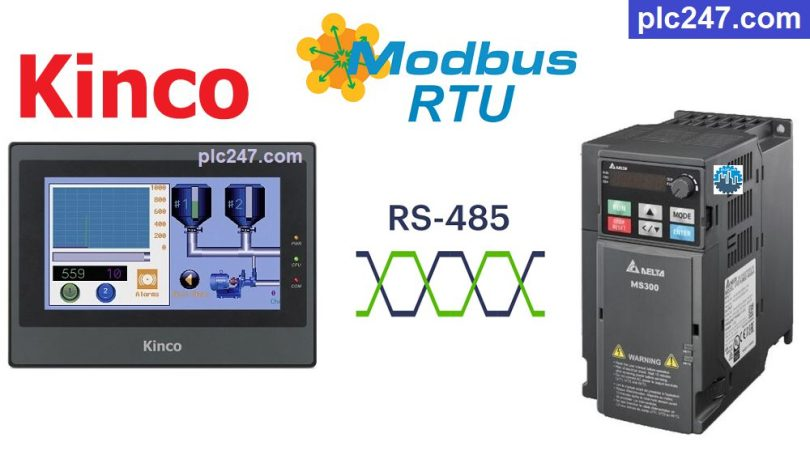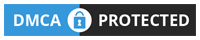# HMI Kinco “Modbus RTU” Delta MS300 TutorialWritten by

Hello friends!
Continuing the series of articles using Kinco HMI to control different devices through Modbus RTU, today plc247.com will guide you to control Delta inverter.

The selected inverter model is Delta MS300, which has a built-in RS485 port to connect to the Kinco HMI monitor.

#### HMI Kinco & Delta MS300 “Connection Diagram”

plc247.com uses the COM.0 port of the MT4414TE HMI (Most other HMIs with a COM.0 port are also RS485 ports)

#### Delta MS300 Modbus Parameters Setting

In addition to the basic parameters of the inverter installed according to the motor (frequency, current, voltage…) we need to install the following communication parameters:

+ P00.20: 1 (Using Modbus Setting Frequency)

+ P00.21: 2 (Using Modbus Control Motor)

+ P09.00: 1 (Slave Address = 1)

+ P09.01: 9.6 (Baudrate = 9600bps)

+ P09.02: 3

+ P09.03: 1.0 (Overtime Com. = 1.0s)

+ P09.04: 14 ( Modbus-RTU 8/E/1 Selection)

##### Note: HMi Kinco Registers Address = “VFD Address (Dec) + 1“

+ Motor Control Address = 2000 (hex) = 8192 (dec) + 1 = 8193 (dec)

• Set Word 8193 = 18 (dec): Forward Run
• Set Word 8193 = 34 (dec): Reverse Run
• Set Word 8193 = 1 (dec): Stop

+ Setting Frequency Address = 2001 (hex) = 8193 (dec) + 1 = 8194 (dec)

• Output Frequency Address = 2103 (hex) = 8451 (dec) + 1 = 8452 (dec)
• Output Current Address = 2104 (hex) = 8452 (dec) + 1 = 8453 (dec)
• Output Volatge Address = 2106 (hex) = 8454 (dec) + 1 = 8455 (dec)

#### HMI Kinco “Modbus RTU” Configuration

We need to set the communication parameters for the Kinco HMI to be the same as those of the Delta MS300 inverter.

### HMI Modbus Programming

+ Main Page Design

+ Frequency Setting Command

+ Run Forward Command

+ Run Reverse Command

+ Stop Command

### Video Tutorial

======

References